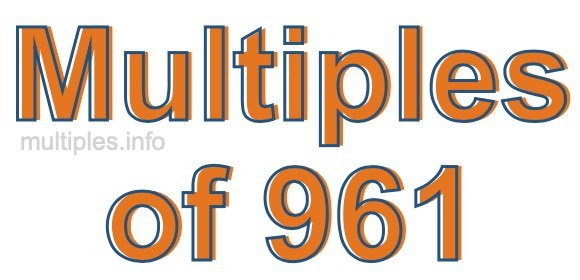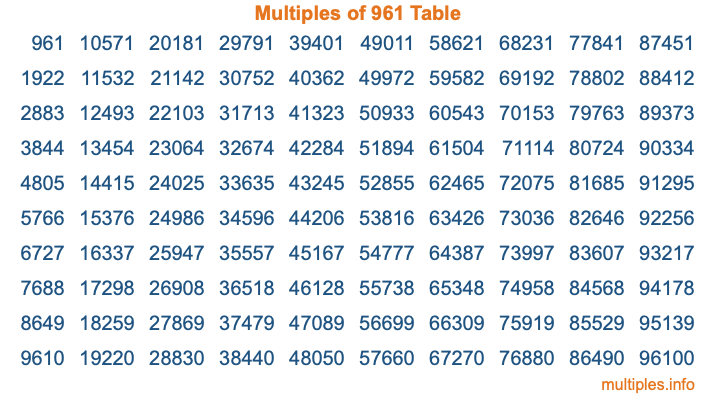Multiples of 961Welcome to the Multiples of 961 page. Here we will first teach you everything you will ever need to know about the multiples of 961, and then give you a study guide summary of everything we taught you to make sure you remember it all. Use this page to look up facts and learn information about the multiples of 961. This page will make you a multiples of nine hundred sixty-one expert!

Definition of Multiples of 961
Multiples of 961 are all the numbers that when divided by 961 equal an integer. Each of the multiples of 961 are called a multiple. A multiple of 961 is created by multiplying 961 by an integer.

Therefore, to create a list of multiples of 961, you start with 1 multiplied by 961, then 2 multiplied by 961, then 3 multiplied by 961, and so on for as long as you want. Thus, the list of the first five multiples of 961 is 961, 1922, 2883, 3844, and 4805. To see a larger list of multiples of 961, see the printable image of Multiples of 961 further down on this page. We also have a category where you can choose any nth multiple of 961.

Multiples of 961 Checker
The Multiples of 961 Checker below checks to see if any number of your choice is a multiple of 961. In other words, it checks to see if there is any number (integer) that when multiplied by 961 will equal your number. To do that, we divide your number by 961. If the the quotient is an integer, then your number is a multiple of 961.

Is  a multiple of 961?

Least Common Multiple of 961 and ...
A Least Common Multiple (LCM) is the lowest multiple that two or more numbers have in common. This is also called the smallest common multiple or lowest common multiple and is useful to know when you are adding our subtracting fractions. Enter one or more numbers below (961 is already entered) to find the LCM.

Check out our LCM Calculator if you need more details about the Least Common Multiple or if you need the LCM for different numbers for adding and subtraction fractions.

nth Multiple of 961
As we stated above, 961 is the first multiple of 961, 1922 is the second multiple of 961, 2883 is the third multiple of 961, and so on. Enter a number below to find the nth multiple of 961.

th multiple of 961

Multiples of 961 vs Factors of 961
961 is a multiple of 961 and a factor of 961, but that is where the similarities end. All postive multiples of 961 are 961 or greater than 961. All positive factors of 961 are 961 or less than 961.

Below is the beginning list of multiples of 961 and the factors of 961 so you can compare:

Multiples of 961: 961, 1922, 2883, 3844, 4805, etc.

Factors of 961: 1, 31, 961

As you can see, the multiples of 961 are all the numbers that you can divide by 961 to get a whole number. The factors of 961, on the other hand, are all the whole numbers that you can multiply by another whole number to get 961.

It's also interesting to note that if a number (x) is a factor of 961, then 961 will also be a multiple of that number (x).

Multiples of 961 vs Divisors of 961
The divisors of 961 are all the integers that 961 can be divided by evenly. Below is a list of the divisors of 961.

Divisors of 961: 1, 31, 961

The interesting thing to note here is that if you take any multiple of 961 and divide it by a divisor of 961, you will see that the quotient is an integer.

Multiples of 961 Table
Below is an image of the first 100 multiples of 961 in a table. The table is in chronological order, column by column. The first column has the first ten multiples of 961, the second column has the next ten multiples of 961, and so on.The Multiples of 961 Table is also referred to as the 961 Times Table or Times Table of 961. You are welcome to print out our table for your studies.

Negative Multiples of 961
Although not often discussed or needed in math, it is worth mentioning that you can make a list of negative multiples of 961 by multiplying 961 by -1, then by -2, then by -3, and so on, to get the following list of negative multiples of 961:

-961, -1922, -2883, -3844, -4805, etc.

Multiples of 961 Summary
Below is a summary of important Multiples of 961 facts that we have discussed on this page. To retain the knowledge on this page, we recommend that you read through the summary and explain to yourself or a study partner why they hold true.

There are an infinite number of multiples of 961.

A multiple of 961 divided by 961 will equal a whole number.

961 divided by a factor of 961 equals a divisor of 961.

The nth multiple of 961 is n times 961.

The largest factor of 961 is equal to the first positive multiple of 961.

961 is a multiple of every factor of 961.

961 is a multiple of 961.

A multiple of 961 divided by a divisor of 961 equals an integer.

961 divided by a divisor of 961 equals a factor of 961.

Any integer times 961 will equal a multiple of 961.

Multiples of a Number
Here you can get the multiples of another number, all with the same attention to detail as we did for multiples of 961 on this page.

Multiples of
Multiples of 962
Did you find our page about multiples of nine hundred sixty-one educational? Do you want more knowledge? Check out the multiples of the next number on our list!